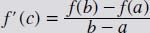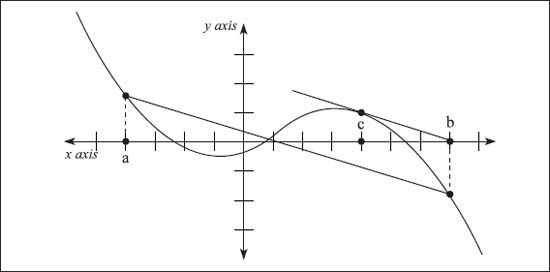# What is the Mean-Value Theorem?

The Mean-Value Theorem has nothing to do with crankiness, but it is one of the most important theoretical tools in the calculus. In written terms, it is defined as the following: If f (x) is defined and continuous on the interval [a, b], and differentiable on (a, b), then there is at least one number on the interval (a, b)—or a < c=""><>—such that:When f(a) = f(b), this is a special case called Rolle’s Theorem, when we know that f (c) will equal zero. Interpreting this, we know that there is a point on (a, b) that has a horizontal tangent.

It is also true that the Mean-Value Theorem can be put in terms of slopes. Thus, the last part of the above equation (on the right of the equal sign) represents the slope of a line passing through (a, f(a)) and (b, f(b)). Thus, this theory states that there is a point c ∈ (a, b), such that the tangent line is parallel to a line passing through the two points.This graph illustrates the concept of the Mean-Value Theorem.

Close

This is a web preview of the "The Handy Math Answer Book" app. Many features only work on your mobile device. If you like what you see, we hope you will consider buying. Get the App